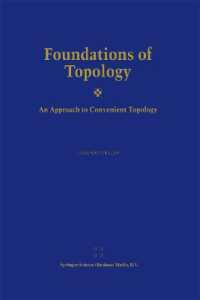### Foundations of Topology : An Approach to Convenient Topology （Reprint）

• 提携先の海外書籍取次会社に在庫がございます。通常2週間で発送いたします。
重要ご説明事項
1. 納期遅延や、ご入手不能となる場合が若干ございます。
2. 複数冊ご注文の場合、分割発送となる場合がございます。
3. 美品のご指定は承りかねます。
• 【重要：入荷遅延について】
各国での新型コロナウィルス感染拡大により、洋書・洋古書の入荷が不安定になっています。
弊社サイト内で表示している標準的な納期よりもお届けまでに日数がかかる見込みでございます。
申し訳ございませんが、あらかじめご了承くださいますようお願い申し上げます。

• 製本 Paperback:紙装版/ペーパーバック版
• 言語 ENG
• 商品コード 9789401039406
• DDC分類 514

### Full Description

A new foundation of Topology, summarized under the name Convenient Topology, is considered such that several deficiencies of topological and uniform spaces are remedied. This does not mean that these spaces are superfluous. It means exactly that a better framework for handling problems of a topological nature is used. In this setting semiuniform convergence spaces play an essential role. They include not only convergence structures such as topological structures and limit space structures, but also uniform convergence structures such as uniform structures and uniform limit space structures, and they are suitable for studying continuity, Cauchy continuity and uniform continuity as well as convergence structures in function spaces, e.g. simple convergence, continuous convergence and uniform convergence. Various interesting results are presented which cannot be obtained by using topological or uniform spaces in the usual context. The text is self-contained with the exception of the last chapter, where the intuitive concept of nearness is incorporated in Convenient Topology (there exist already excellent expositions on nearness spaces).

### Contents

Preface. Introduction. 0. Preliminaries. 1. Topological constructs. 2. Reflections and coreflections. 3. Topological universes. 4. Completions of semiuniform convergence spaces. 5. Connectedness properties. 6. Function spaces. 7. Relations between semiuniform convergence spaces and merotopic spaces (including nearness spaces). Appendix: Some algebraically topological aspects in the realm of Convenient Topology. Exercises. Implication scheme for various SUConv-invariants. Table: Preservation properties of some SUConv-invariants. Diagram of relations between various subconstructs of SUConv (including their relations to merotopic and nearness spaces). Bibliography. List of axioms. List of symbols. Index.